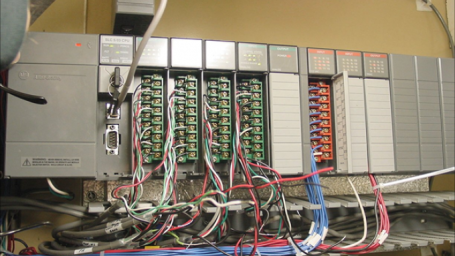Interactive

# Introduction to Programmable Logic Controllers (PLC)

Martech
Updated Jan 21, 2021

Upon completing this lesson, you will be able to describe the design and function of the major components of a PLC. In addition, you will be able to convert numbers from decimal to binary, binary coded decimal, and hexadecimal. • Describe the purpose of each of the major PLC components • Discuss basic PLC operation • Describe the internal structure of the CPU • Describe the internal architecture of a typical PLC • Identify typical input and output devices • Discuss the function of the I/O (input/output) modules • Identify types of signals used by PLC and discuss how they are handled • Define ladder logic and explain its use • Discuss how binary, octal, and hexadecimal numbering systems are used in PLC programming

;